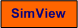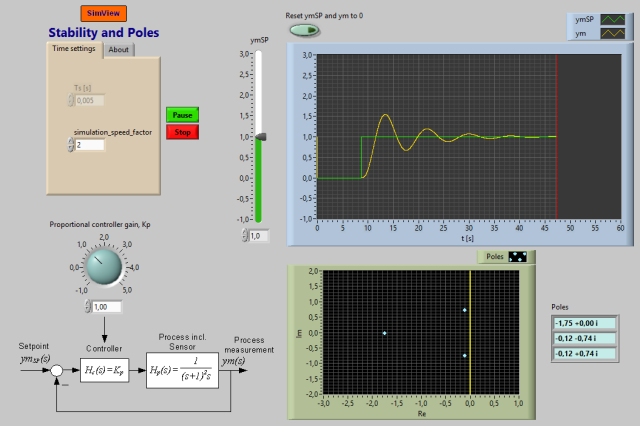# Poles and Stability of Feedback System

Snapshot of the front panel of the simulator:## Description of the simulated system

In this simulator a feedback system, namely a control system, is simulated. The controller is a P controller. The front panel of the simulator shows a block diagram of the control system.

It can be shown that the transfer funcion from setpoint ymSP to process measurement ym is

ym(s)/ymSP(s) = [Hc(s)Hp(s)]/[1 + Hc(s)Hp(s)]

= Kp/(s3+2s2 + s + Kp)

= Kp/a(s)

where a(s) is the characteristic polynomial.

The stability property of the feedback system can be determined from the poles, which are the roots of the characteristic equation:

a(s) = s3+2s2 + s + Kp = 0

In the simulator the controller gain Kp can be adjusted in real time, and the response in the process measurement and the poles are shown.

## Aims

The aim of this simulator is to observe the connection between stability and pole placement.